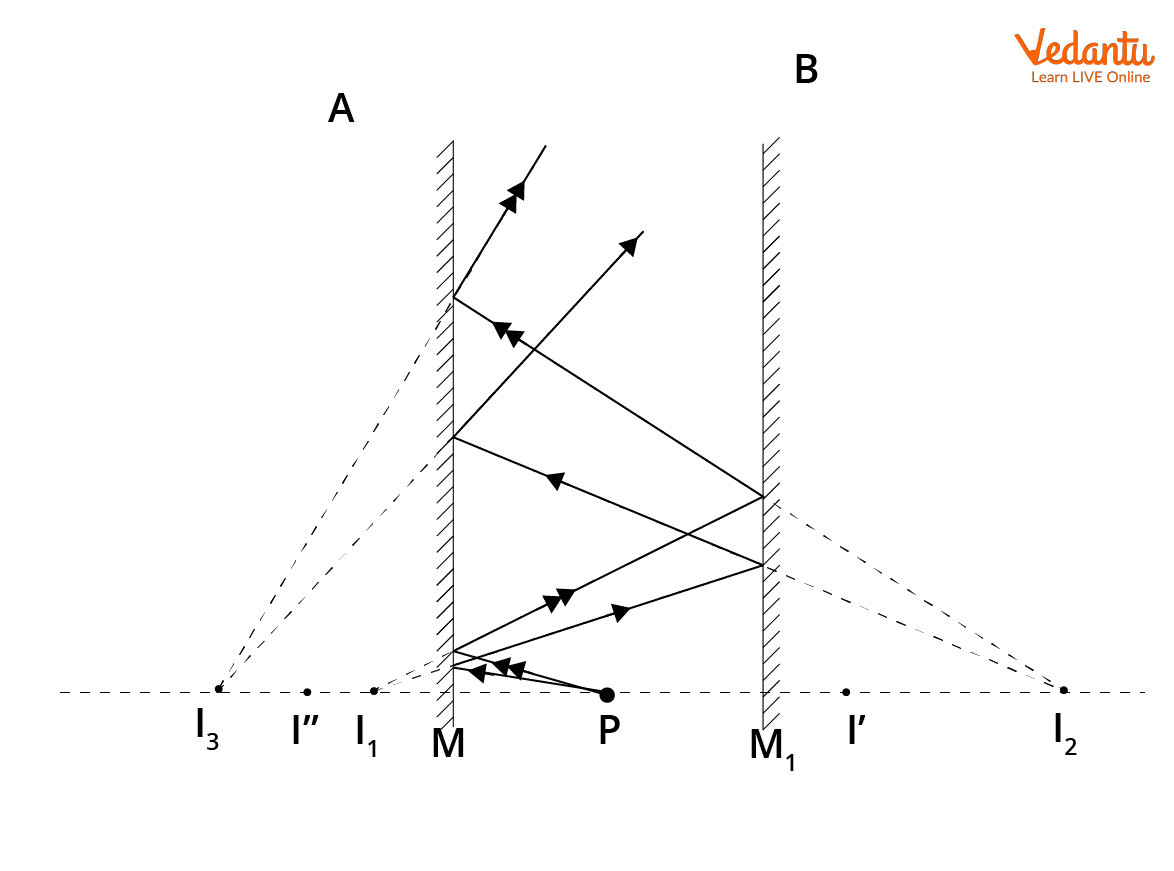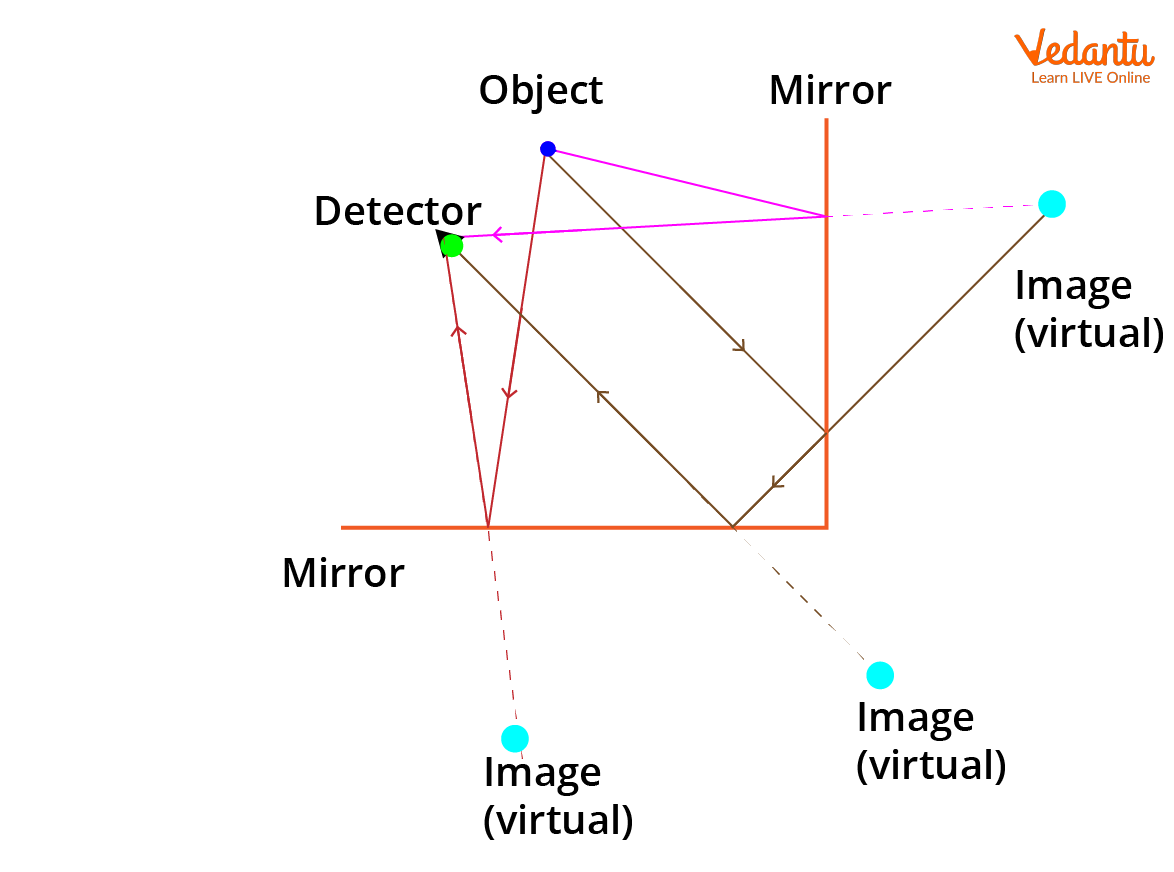Courses
Courses for Kids
Free study material
Free LIVE classes
More

# Number of Images Formed by Two Plane Mirrors | JEE## An Introduction to Images Formed by Two Plane Mirrors

The images formed by two plane mirrors depend on the angle at which they are placed to one another. Two plane mirrors can be placed in parallel or perpendicular to one other. As images are formed on the angle between two plane mirrors, when the angle between the mirrors increases, the images formed decrease and vice versa.  Plane mirrors have some interesting properties, which include lateral inversion. Another interesting property of it is the ability to form multiple reflections. Let us understand these concepts thoroughly.

## What are Plane Mirrors?

Plane mirrors are nothing but a flat reflective surface formed by an added layer of silver nitrate or aluminium. The image formed by the plane mirrors is erect and is formed by two laws of reflection. These are:

1. Incident ray, reflected ray and the normal all lie on the same plane

2. The angle of incidence is equal to the angle of reflection.

Plane mirrors are all around us. We can find them in offices, schools at our homes and so on. Thus, plane mirrors are used in a plethora of day-to-day applications. When an object is placed in front of a plane mirror the light rays incident on it are either absorbed, transmitted or reflected. The light rays that are reflected predominately are the ones that form an image.

## Image Formed by a Plane Mirror

The images formed by a plane mirror can be infinite or of a finite number. The infinite or the formation of multiple reflections formed by a plane mirror is achieved by using two plane mirrors in parallel.Formed by Two plane mirrors

The orientation of the two plane mirrors can also be changed. The two mirrors can also be placed at 90 degrees to one another. In such cases, the images formed are usually of a finite number.Formed by plane mirrors placed at 90 degrees

## Plane Mirror Image Formula

The plane mirror image formula or the formula of number of images formed by two mirrors is given as,

$n=\dfrac{360}{\theta}-1$

Where $\theta$ is the angle between the two mirrors and n is the number of images formed.

Let us understand this with an example. Let’s assume that the plane mirrors are placed at 90 degrees to one another. Then $\theta$ would be 90 degrees. Substituting this in the formula $n=\dfrac{360}{\theta}-1$

We have:

$n=\dfrac{360}{90}-1$

$\Rightarrow n=4-1$

$\therefore n=3$

Thus, the images formed here are 3.

Let us now take another example wherein the angle between the two plane mirrors is 60 degrees. Substituting this in the above formula i.e,

$n=\dfrac{360}{\theta}-1$

We get:

$n=\dfrac{360}{60}-1$

$\Rightarrow n=6-1$

$\therefore n=5$

The images formed in this case will be 5.

Thus, it is understandable from above that angle between mirrors is fundamental to get the desired number of images, since this entails the usage of plane mirrors in different applications. Ever wondered why periscopes employ plane mirrors? Plane mirrors in periscopes have the ability to change the direction of light rays, which makes the object viewable to the person viewing from the submarine.

Periscopes are usually employed when we are not able to see the object on the other side or beyond any obstruction. Kaleidoscope is another interesting application of plane mirrors. Kaleidoscopes form multiple reflections. When we look inside a kaleidoscope, several patterns are seen. This is due to plane mirrors used in them.

## Conclusion

The article above talks about the number of images formed by two plane mirrors. The orientation of the two mirrors can be perpendicular or placed in parallel to one another. When the two mirrors are placed in parallel, plane mirrors form multiple reflections of an object. This is an interesting application of plane mirrors that is used in kaleidoscopes. The different angles between two plane mirrors is also discussed. The formula of the number of images by two mirrors is given above and is illustrated using different examples. Thus, the articles clearly discusses the various placement orientation of two mirrors, followed by their application and characteristics.

## FAQs on Number of Images Formed by Two Plane Mirrors | JEE

1. What are some characteristics of plane mirrors?

Some of the characteristics of a plane mirror are:

• It forms a virtual and erect image.

• The image formed is of the same size as the object.

• The image formed will be at the same distance from the mirror as the distance between the object and the mirror.

• Additionally, the images formed by a plane mirror are laterally inverted. Lateral inversion is an interesting phenomenon. In lateral inversion, the images formed will be inverted left to right. However, the same is not true for images to be inverted from top to bottom.

2. State a few applications of plane mirrors?

Some of the applications of plane mirrors are that they are used in periscopes, kaleidoscopes, solar cookers, dental use, dressing mirrors and so on. The application of plane mirrors in solar cookers is an interesting application. As plane mirrors reflect most of the light rays falling on it, the reflected rays can be used to cook food in an efficient way. This saves energy and is thus a renewable way to cook food. Plane mirrors are also used by some people for security purposes. Security officials use plane mirrors to get an image of the beneath of a car to detect any explosives installed. Plane mirrors can also be used to avoid accidents at blind turns. These mirrors are installed at roadsides with blind turns that are exposed to a huge amount of traffic.